# Optimal trajectory

(diff) ← Older revision | Latest revision (diff) | Newer revision → (diff)

A curvein an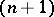-dimensional space of variablesalong which a point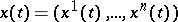, whose motion is determined by the vector differential equation(1)

is transferred from its original position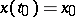(2)

to a final position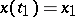(3)

under the influence of an optimal control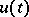which minimizes a given functional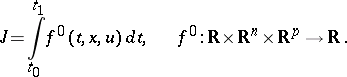(4)

The choice of an optimal control is subject to the restriction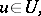(5)

whereis a closed set of permissible controls,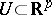. The initial and final moments of time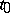and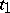are assumed to be fixed and free, respectively.

An optimal trajectory is defined in the same way for variational problems of a more general type than (1)–(5), for example, for problems with movable end-points and with constraints on the phase coordinates. For methods of tracing optimal trajectories, see Variational calculus, numerical methods of.

For autonomous problems, in which the functions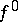,do not explicitly depend on the time: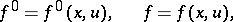the concept of a phase optimal trajectory proves to be more apt for the theory and its applications. A phase optimal trajectory is the projection of an optimal trajectory onto the-dimensional subspace of phase variables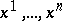. For autonomous problems, a phase trajectory does not depend on the choice of the initial moment of time.

Research into the set of phase optimal trajectories which transfer the system from an arbitrary initial position to a given final position (or from a given initial position to an arbitrary final one) enables one to answer many qualitative questions arising from the variational problem being considered. The formation of the set of phase optimal trajectories is a compulsory step in the construction of a synthesis of an optimal feedback control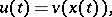which ensures a movement along an optimal trajectory at any point in the phase space.

How to Cite This Entry:
Optimal trajectory. Encyclopedia of Mathematics. URL: http://encyclopediaofmath.org/index.php?title=Optimal_trajectory&oldid=13714
This article was adapted from an original article by I.B. Vapnyarskii (originator), which appeared in Encyclopedia of Mathematics - ISBN 1402006098. See original article